# Simulation of the beam loading for the CLIC

• Slides: 26
Download presentationSimulation of the beam loading for the CLIC accelerating structures Oleksiy Kononenko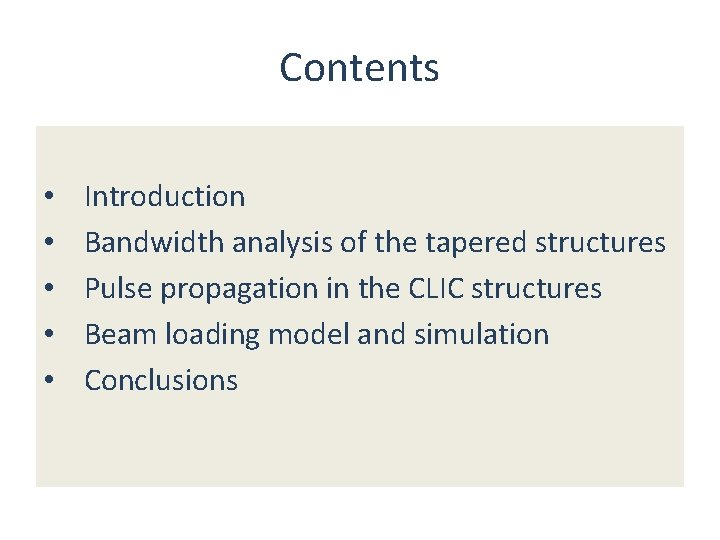Contents • • • Introduction Bandwidth analysis of the tapered structures Pulse propagation in the CLIC structures Beam loading model and simulation Conclusions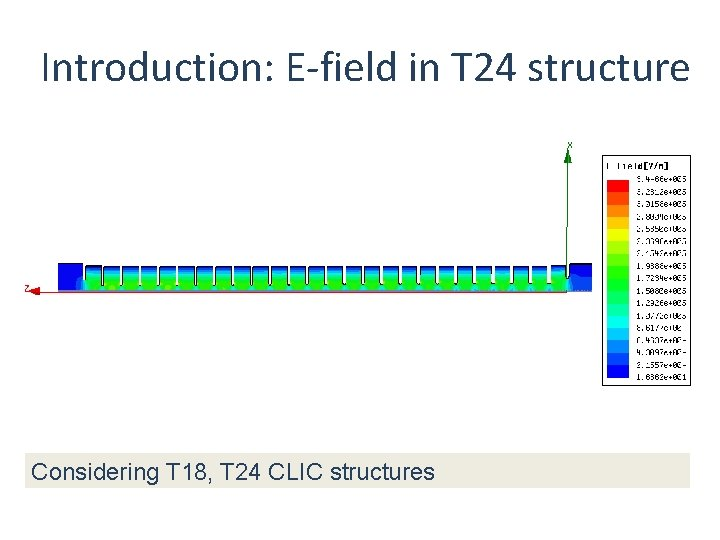Introduction: E-field in T 24 structure Considering T 18, T 24 CLIC structures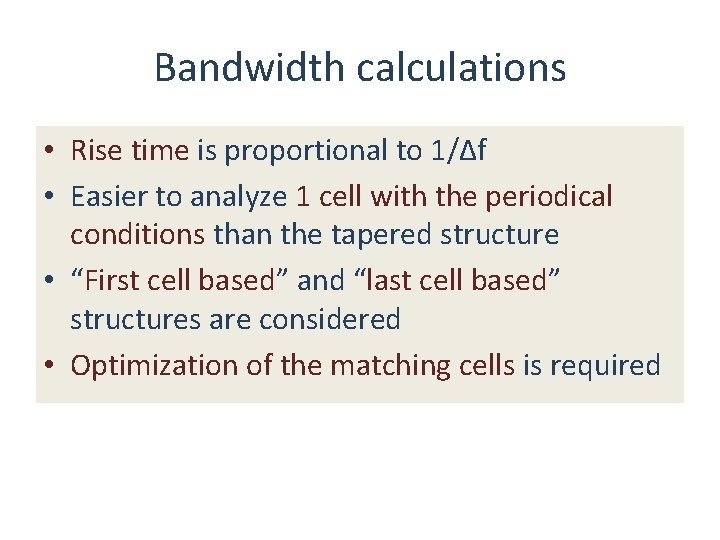Bandwidth calculations • Rise time is proportional to 1/Δf • Easier to analyze 1 cell with the periodical conditions than the tapered structure • “First cell based” and “last cell based” structures are considered • Optimization of the matching cells is required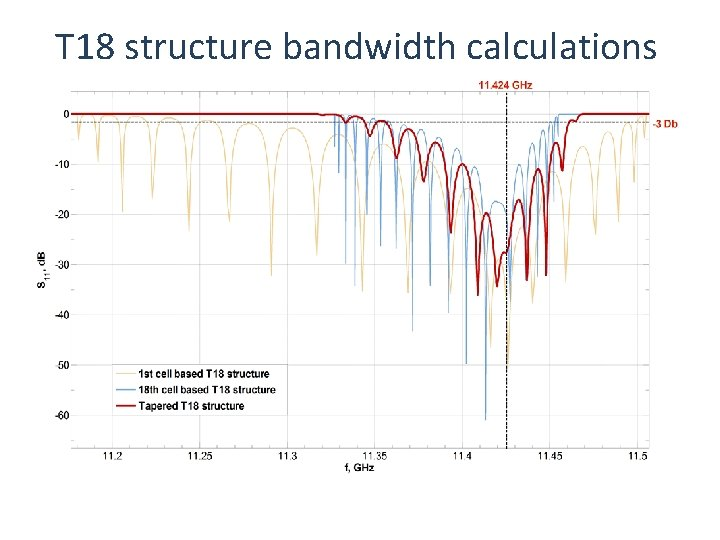T 18 structure bandwidth calculationsT 24 structure bandwidth calculations t rise ~ 1 / BWS-parameters in T 24 structure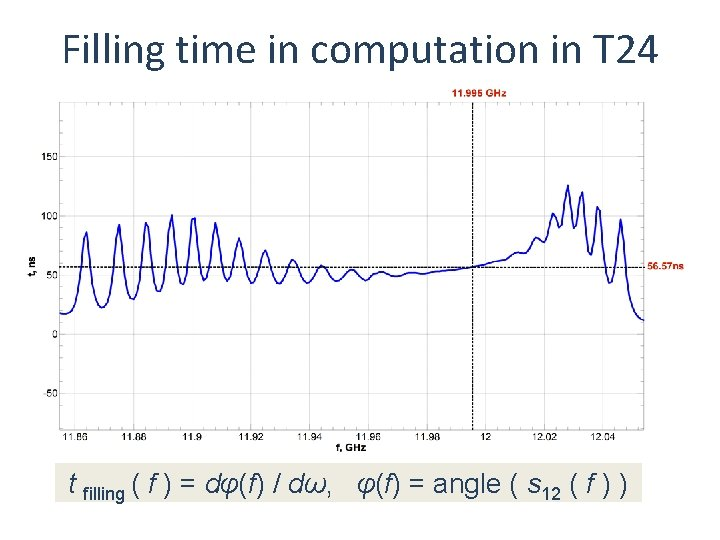Filling time in computation in T 24 t filling ( f ) = dφ(f) / dω, φ(f) = angle ( s 12 ( f ) )Rectangular pulse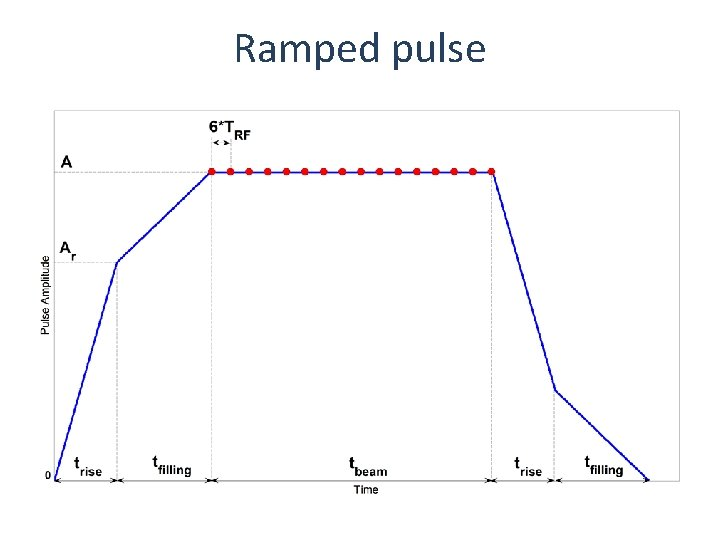Ramped pulseRectangular pulse propagation in T 24 s 12(f) → [ ift ] → s 12 (t) → [conv prect(t)] → pout(t)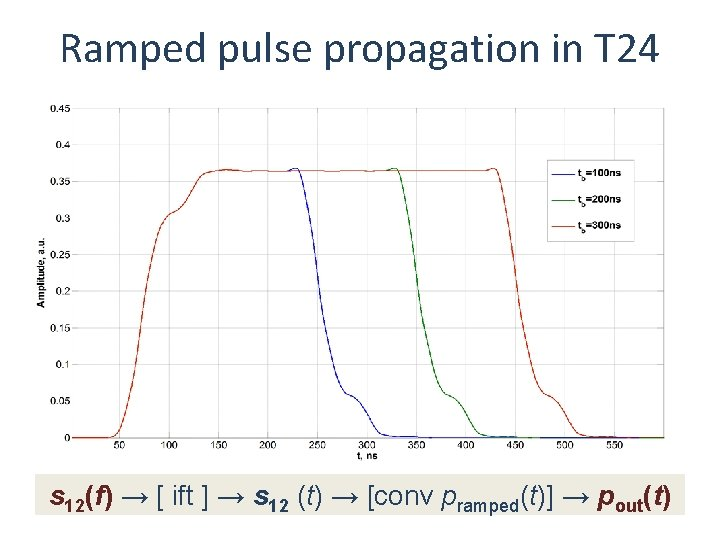Ramped pulse propagation in T 24 s 12(f) → [ ift ] → s 12 (t) → [conv pramped(t)] → pout(t)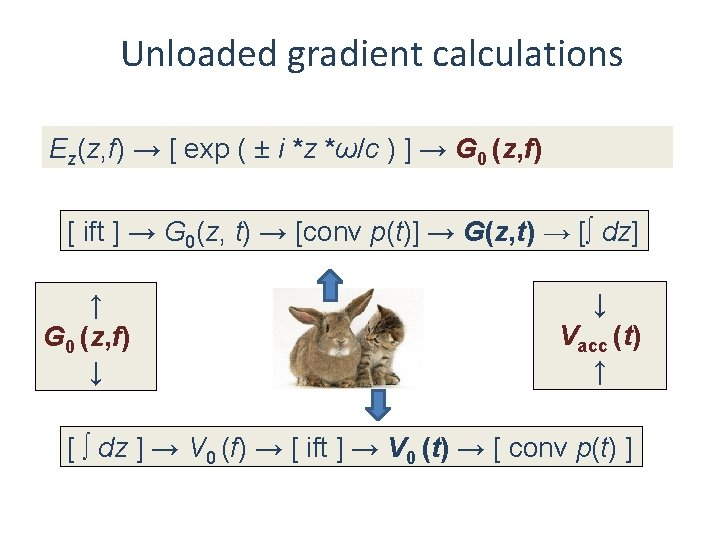Unloaded gradient calculations Ez(z, f) → [ exp ( ± i *z *ω/c ) ] → G 0 (z, f) [ ift ] → G 0(z, t) → [conv p(t)] → G(z, t) → [∫ dz] ↑ G 0 (z, f) ↓ ↓ Vacc (t) ↑ [ ∫ dz ] → V 0 (f) → [ ift ] → V 0 (t) → [ conv p(t) ]Unloaded Vacc in T 24 structure x 10 7 9 Accelarating Voltage, arbitrary units V V+ 8 V 7 6 5 4 3 2 1 0 -1 11. 7 11. 8 11. 9 12 f, GHz 12. 1 12. 2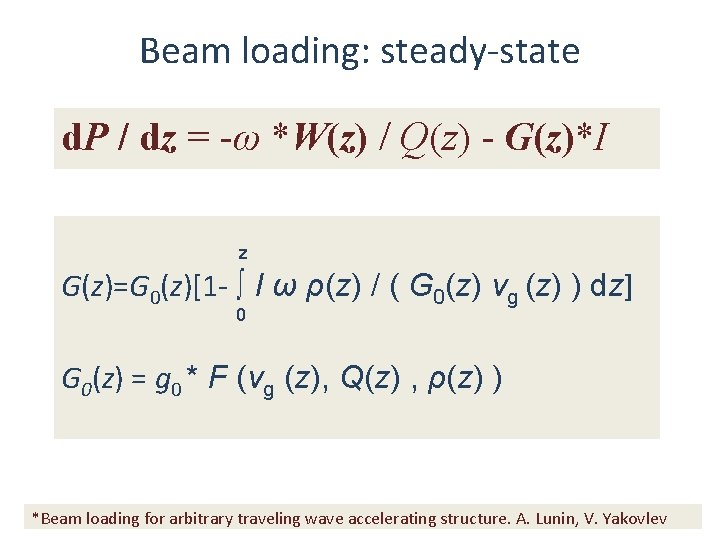Beam loading: steady-state d. P / dz = -ω *W(z) / Q(z) - G(z)*I z G(z)=G 0(z)[1 - ∫ I ω ρ(z) / ( G 0(z) vg (z) ) dz] 0 G 0(z) = g 0 * F (vg (z), Q(z) , ρ(z) ) *Beam loading for arbitrary traveling wave accelerating structure. A. Lunin, V. Yakovlev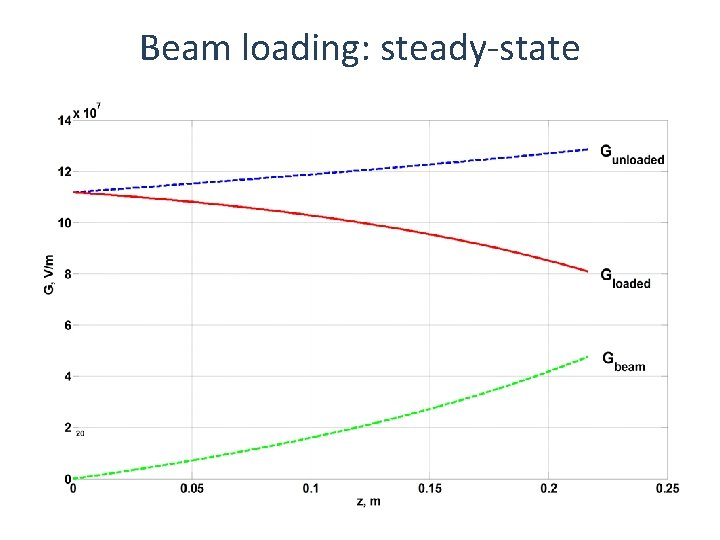Beam loading: steady-state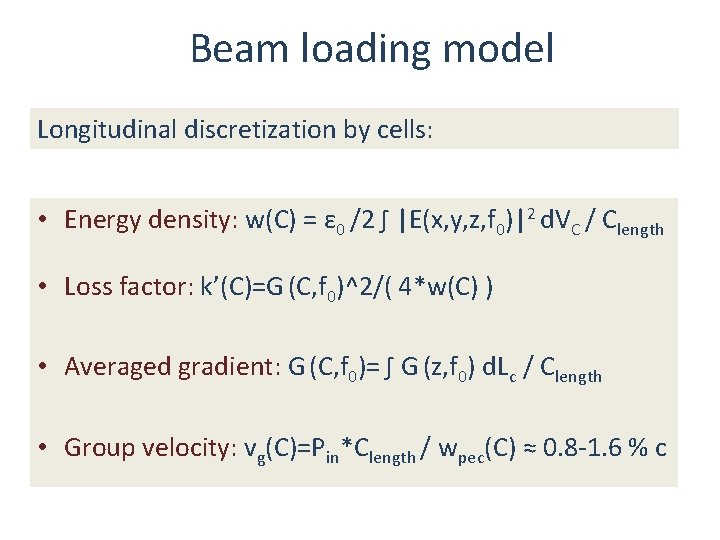Beam loading model Longitudinal discretization by cells: • Energy density: w(C) = ε 0 /2 ∫ |E(x, y, z, f 0)|2 d. VC / Clength • Loss factor: k’(C)=G (C, f 0)^2/( 4*w(C) ) • Averaged gradient: G (C, f 0)= ∫ G (z, f 0) d. Lc / Clength • Group velocity: vg(C)=Pin*Clength / wpec(C) ≈ 0. 8 -1. 6 % c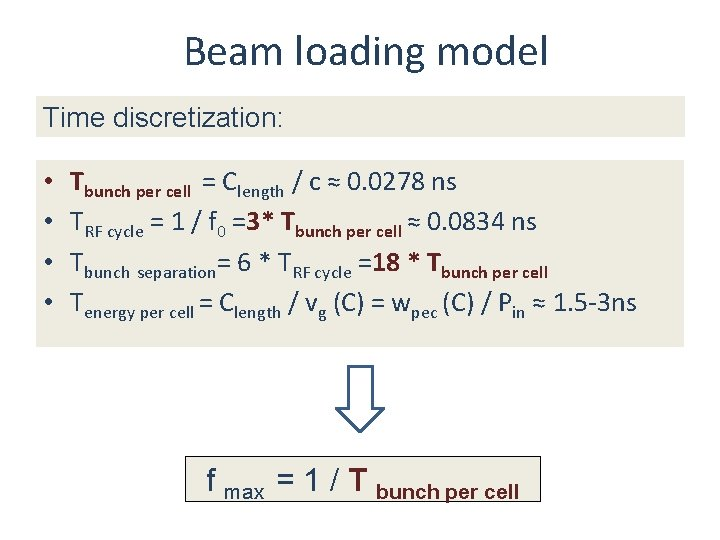Beam loading model Time discretization: • • Tbunch per cell = Clength / c ≈ 0. 0278 ns TRF cycle = 1 / f 0 =3* Tbunch per cell ≈ 0. 0834 ns Tbunch separation= 6 * TRF cycle =18 * Tbunch per cell Tenergy per cell = Clength / vg (C) = wpec (C) / Pin ≈ 1. 5 -3 ns f max = 1 / T bunch per cellBeam loading model • Energy lost per bunch per cell: Wbunch(t, C)=k’(C)* q 2(t, C) * Clength Wfield(t, C) =G(C)* q(t, C) * Clength • Energy moves with vg(C) • Wall losses Pwalls(C) = ω*W(C) / Q(C) • Total energy W(t, C) = ∑ Wb(t, C) • Gradient: G(t, C)=2*sqrt(k’(C) * W(t, C) / Clength)Beam loading simulation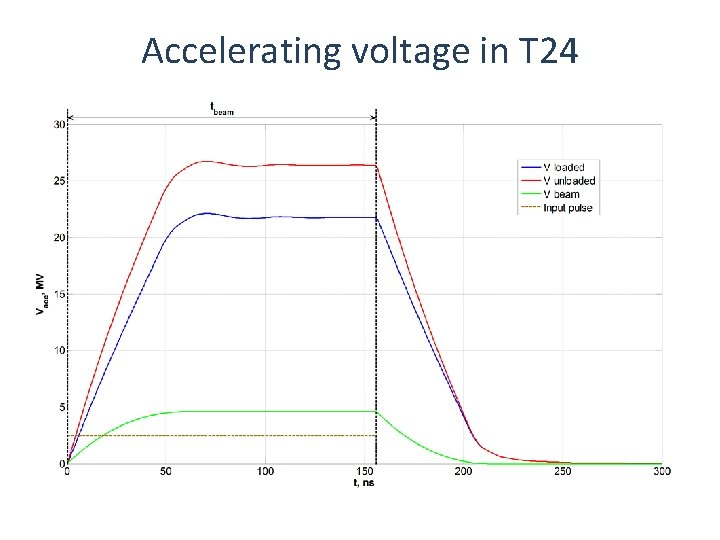Accelerating voltage in T 24Accelerating voltage in T 24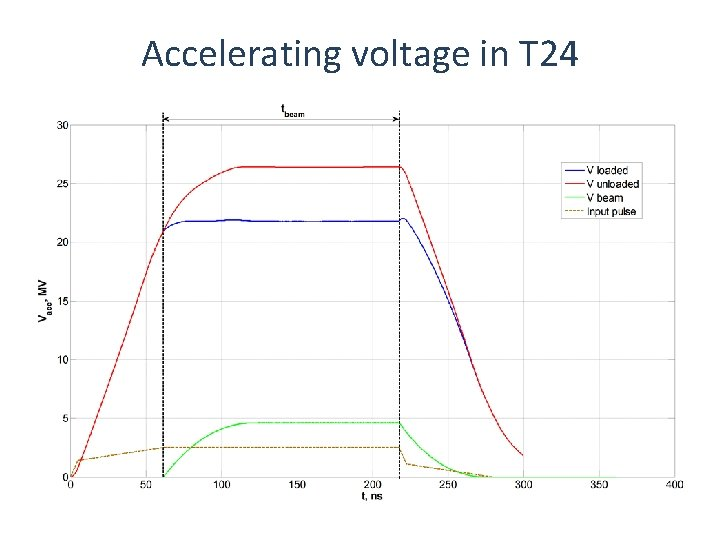Accelerating voltage in T 24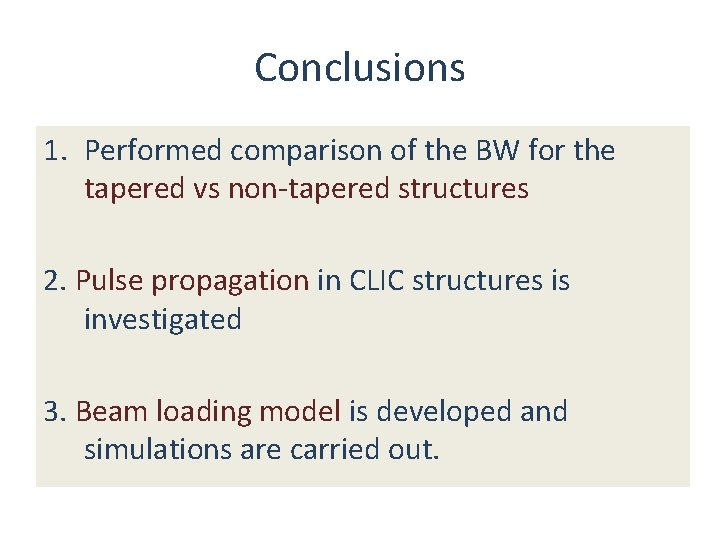Conclusions 1. Performed comparison of the BW for the tapered vs non-tapered structures 2. Pulse propagation in CLIC structures is investigated 3. Beam loading model is developed and simulations are carried out.Further steps 1. Optimization of the pulse shape 2. More accurate beam loading calculationsThank you for the attention!